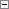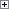The following functions are used to filter records in a table:

• SETCURRENTKEY
• SETRANGE
• SETFILTER
• GETRANGEMIN
• GETRANGEMAX

These functions are some of the most commonly used C/AL functions. They set limits on the value of one or more specified fields, so that only a subset of the records are displayed, modified, deleted, and so on.

SETCURRENTKEY Function

SETCURRENTKEY selects a key for a record and sets the sort order that is used for the table in question.

SETCURRENTKEY has the following syntax.Copy Code
[Ok :=] Record.SETCURRENTKEY(Field1, [Field2],...)

When you use SETCURRENTKEY the following rules apply:

• Inactive fields are ignored.
• When searching for a key, the first occurrence of the specified fields is selected. This means the following:
• If you specify only one field as a parameter when you call SETCURRENTKEY, the key that is actually selected may consist of more than one field.
• If the field that you specify is the first component of several keys, the key that is selected may not be the key that you expect.
• If no keys can be found that include the fields that you specify, the return value is FALSE. If you do not test the return value, a run-time error occurs. If you do test the return value, the program will continue to run even though no key was found.

SETRANGE Function

SETRANGE sets a simple filter on a field.

SETRANGE has the following syntax.Copy Code
Record.SETRANGE(Field [,From-Value] [,To-Value]);

In the following example, SETRANGE filters the Customer table by selecting only those records where the No. field has a value between 10000 and 90000.Copy Code
Customer.SETRANGE("No.",'10000','90000');

When you use SETRANGE the following rules apply:

• SETRANGE removes any filters that were set previously and replaces them with the From-Value and To-Value parameters that you specify.
• If you use SETRANGE without setting the From-Value and To-Value parameters, the function removes any filters that are already set.
• If you only set the From-Value, the To-Value is set to the same value as the From-Value.

SETFILTER Function

SETFILTER sets a filter in a more general way than SETRANGE.

SETFILTER has the following syntax.Copy Code
Record.SETFILTER(Field, String [, Value], ...]);

Field is the name of the field on which you want to set a filter. String is the filter expression. String may contain placeholders, such as %1 and %2, to indicate where to insert the Value parameter(s) in a filter expression.

The following example selects records where the value of No. is larger than 10000 and not equal to 20000.Copy Code
Customer.SETFILTER("No.", '>10000 & <> 20000');

If the variables Value1 and Value2 have been assigned "10000" and "20000" respectively, then you can use the following statement to create the same filter.Copy Code
Customer.SETFILTER("No.",'>%1&<>%2',Value1, Value2);

GETRANGEMIN Function

GETRANGEMIN retrieves the minimum value of the filter range that is currently applied to a field.

GETRANGEMIN has the following syntax.Copy Code
Record.GETRANGEMIN(Field);

A run-time error occurs if the filter that is currently applied is not a range. For example, you can set a filter as follows.Copy Code
Customer.SETFILTER("No.",'10000|20000|30000');

With this filter, the following code fails because the filter is not a range.Copy Code
BottomValue := Customer.GETRANGEMIN("No.");

GETRANGEMAX Function

GETRANGEMAX retrieves the maximum value of the filter range that is currently applied to a field.

GETRANGEMAX has the following syntax.Copy Code
Value := Record.GETRANGEMAX(Field)